Maths
Study Material

# Permutation and Combination – Basics – Introduction

Fundamental of counting, permutations and combinations are covered in this blog. Start you journey into the world of combinatorics by learning the basics. Few theorems included in the blog.

5 minutes long
Posted by Tanwir Silar, 11/8/2021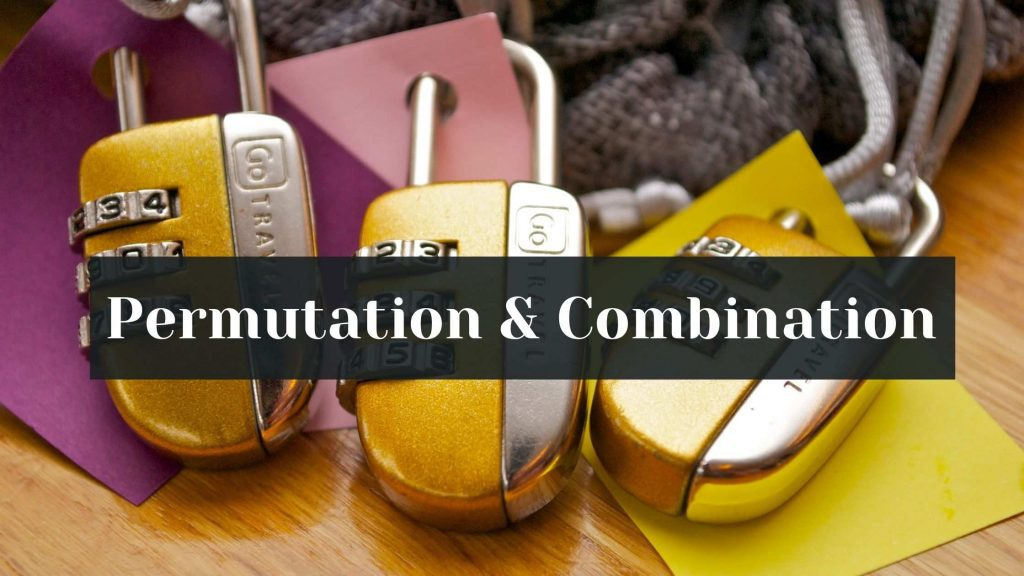Hesap Oluştur

Got stuck on homework? Get your step-by-step solutions from real tutors in minutes! 24/7. Unlimited.

In this blog, learn about the fundamental principle of counting and how to count the number of permutation and combination in a easier manner.

## Fundamental Principle of Counting

There are two rules of counting. The multiplication rule and the addition rule.

## Multiplication rule

For a task to be completed, two events A, B need to be performed. If the event A can occur in m different ways, following which event B can occur in n different ways, then the total number of ways in which task can be completed is m x n.
For instance, the number of ways a person can dress who has 3 pants and 2 shirts is 3 x 2 = 6.

For a task to be completed, either of the two events A, B should be performed, but not both. If the event A can occur in m different ways, and event B can occur in n different ways, then the total number of ways to complete the task is m+n.

## What is a Permutation?

A permutation is an arrangement in a definite order of a number of objects taken some or all at a time. The number of permutations of n different objects taken r at a time, when repetition is not allowed is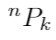, when repetition is allowed is nk.

## What is a Combination?

A combination is an arrangement in which the order of elements arranged doesn’t matter. The number of combinations of n different objects taken r at a time, when repetition is allowed is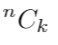, when repetition is not allowed is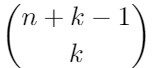.

## Factorial notations

Factorials come handy to calculate permutation and combination. Mainly because,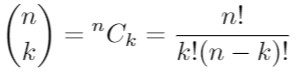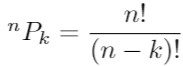.
Getting familiar with factorial notation comes in handy while solving problems of Permutation and Combination.

## Permutation and Combination Theorem 1

Theorem 1:
Let there are n objects. In these n objects we have p1 objects of one kind, p2 objects of second kind, … pk objects of kth kind and the rest if any, are of different kind. Then the number of permutations of the n objects taken all at a time equals to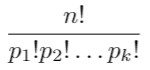## Permutation and Combination Theorem 2

Theorem 2:
This theorem tells us how permutation and combination of n different objects taken r at a time, when repetition is not allowed are related as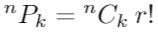This theorem tells us that to find the number of permutations we can find the number of combinations and multiply it with r! ( use the result carefully, as certain conditions apply).

Example:
Find the number of combinations and permutations of choosing 4 cards out of a 52 card deck?
As discussed earlier, the number of combinations are 52 choose 4 =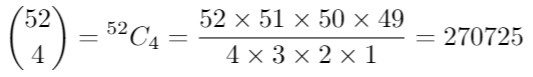Using the above theorem, we could easily get the number of permutations from number of combinations.
In continuation, the number of permutations are =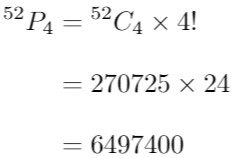We hope, this blog(permutation and combination) was helpful.